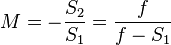Thin Lens Equations

This has been turning my head inside out for days, so i’m going to write it down.

There are a set of simple equations for describing the behaviour of lenses. One of these is an approximation that can used when working with thin lenses, and is called, drum roll, the thin lens equation! It looks like this:where  f is the focal length, and S1 and S2 are the distances between the object, the real image, and the lens:This means that with the lens focused at infinity we can simplify things down to:

1/∞ + 1/s2 = 1/40

0 + 1/s2 = 1/40

∴ s2 = 40

And with the lens focused at it’s closest, 500mm:

1/500 + 1/s2 = 1/40

s2 = 1/(1/40 – 1/500)

∴ s2 = 43.47

Which implies that the lens moves 3.47mm further away when focused at it’s closest.

Having got this far, we can now do something stupid and try to calculate what happens when you add an extension tube of 32mm between the lens and the camera.

1/s1 + 1/(40 + 32) = 1/40

s1 = 1/(1/40 – 1/72)

∴ s1 = 90

Which implies that, with the extension tube, the furthest object we can focus on is 90mm. And further:

1/s1 = 1/(40 + 32 + 3.47) = 1/40

s1 = 1/(1/40 – 1/75.47)

∴ s1 = 85.1

These numbers look completely crazy to me, but what they imply is that the distance between being focused at infinity, and focused at 500mm, is reduced to 4.9mm.

Also, i don’t see how to relate these number to the physical camera… mostly because a Distagon 40mm lens with something like 13 elements is just about as far from a “thin lens” as it’s possible to get without building a space telescope.

Regardless, if i haven’t screwed up my maths, the implication is that the distance within which it is possible to focus is really really slim, and that does at least match my experience with physical camera.

Tell me if it’s screwed up.

But more:

There’s another part to this that is also interesting… the wikipedia page goes onto say that the magnification of the lens (the size of the real image relative to the size of the object is simply the ratio of S2 / S1.Plugging in the numbers above for when lens is focused at it’s closest you get a magnification of roughly 113%. This is pretty hard to guess at through the viewfinder, but thinking about how much of my diving watch filled the frame, it might be close to correct.